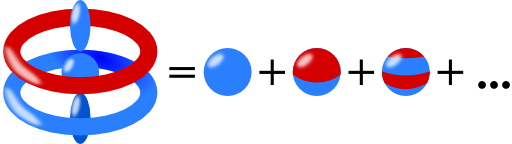## Multipole Moments in Rotational SymmetryIn rotational symmetry, the multipole moments of a charge distribution are described by just one component per order $$l$$. This is a drastic difference to the usual $$2l+1$$ independent components. Get to know this simplifications with two examples and derive it for any charge distribution with rotational symmetry!

## Problem StatementA charge distribution shall obey rotational symmetry with respect to the $$z$$ axis. Proof that its multipole moments are entirely given by its components with respect to the axis of symmetry. So, the dipole moment just depends on its $$z$$ component $$p_{z}$$, the quadrupole moment on $$Q_{zz}$$. and so on. Let us approach this very useful conclusion in two steps:

1. Examples:

a) Show that the dipole moment is given by $$\mathbf{p}=p_{z}\mathbf{e}_{z}$$.
b) Calculate that the cartesian quadrupole tensor is diagonal and that $$Q_{xx}=Q_{yy}=-\frac{1}{2}Q_{zz}$$ holds.

2. Proof of the general conclusion:

a) Derive that the general solution of the electrostatic potential outside of the charge distribution is given by$\phi\left(r,\theta\right) = \frac{1}{4\pi\varepsilon_{0}}\sum_{l=0}^{\infty}\frac{b_{l}P_{l}\left(\cos\theta\right)}{r^{l+1}}$using a separation of variables in spherical coordinates. Note that the so-called Legendre Polynomials $$P_{l}\left(\cos\theta\right)$$ are given by the differential equation $\frac{1}{\sin\theta}\frac{d}{d\theta}\sin\theta\frac{d}{d\theta}P_{l}\left(\cos\theta\right)+l\left(l+1\right)P_{l}\left(\cos\theta\right) = 0\ ,$ where only a solution exists if $$l\in\mathbb{N}_{0}=\left\{ 0,\ 1,\ 2,\ \dots\right\}$$.
b) Use $$P_{l}\left(\cos\theta=1\right)=1\ \forall l$$ and $\frac{1}{\sqrt{1-2\xi\cos\theta+\xi^{2}}} \equiv \sum_{l=0}^{\infty}P_{l}\left(\cos\theta\right)\xi^{l}$to show that the multipole moments are given by$b_{l} = \int P_{l}\left(\cos\theta^{\prime}\right)r^{\prime l}\rho\left(r^{\prime},\theta^{\prime}\right)dV^{\prime}\ .$